## Sunday, May 24, 2020

RE-231
SEE 2075(2019)
Optional Mathematics
Time: 3hrs                                                                         Full Marks: 100
Group ‘A’ [8x (2+ 2) = 32]
1.
a. If f(x) = 2x - 1 then find the value of ff (-1).
b. If a factor of a polynomial p(x) = 2x3 - 6x2 - 5m - 2 is x - 2, find the value of m.
2.
a. Calculate the arithmetic mean and geometric mean between 4 and 16.
b. Write the definition of inverse matrix. In which condition the inverse matrix cannot be defined? Write it.
3.
a. If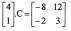find the matrix of C.
b. Write down the formula to find the angle between the lines y = m1 x + c1 and y = m2 x + c2. Also write the condition of perpendicularity of these lines.
4.
a. If the lines represented by 16x2 – kxy + 9y2 = 0 are coincident, find the value of k.
b. The end points of a diameter of a circle are (O, 6) and (8, O). Find the equation of the circle.
5.
a. Find the value of sin1650 without using a trigonometric table or a calculator.
b. If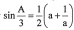, prove that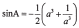.
6.
a. Prove that: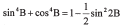b. Solve: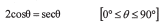7.
a. Ifunit and the angle betweenis 300, find the length.
8.
a. Find the coordinates of the image of a point A (3, -5) after reflection on the line x + y = 0 followed by the rotation through +900 about the origin.
b. Find the transformation represented by the matrix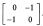Group ‘B’ [17x4= 68 ]
9. If g(x)= 2x -3 andfog(x) = 6x -11, then find f-1(x).
10. Solve: 2x3 - 9x2 + 7x + 6 = O.
11. The product of the first five terms of a geometric series is 243. If the third term of the geometric series is equal to the tenth term of an arithmetic series, find the sum of the first 19 terms of the arithmetic series.
12. Solve graphically the quadratic equation x2 - 3x = 10.
13. Solve by matrix method: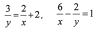14. Find the equation of any one straight line passing through the point(4, -1) and making an angle of 450 with the line 2x -3y = 5.
15. If an angle between the pair of lines represented by the equation 2x 2 + + 3y2 = O is 450, then find the positive value of k and also find the separate equations of the lines.
16. In the given figure, the circle A with centre X passes through the centreY of the circle B. If the equation of circle B is x2 + Y 2 -4x + 6y - 12 and the co-ordinates of X are (-4, 5), then find the equation of the circle A.17. Without using the calculator or table, find the value of:
sin 1000.sin1200.sin1400.sin160 0
18. If P, Q and R are the angles of a ΔPQR, prove that.
19. Solve: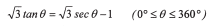20. From the roof and foot of a house, the angles of depression and elevation of the top of a tree are 600 and 300 respectively. If the height of the tree is 15ft, find the height of the house.
21. Prove by vector method that the circumference angle ACB of a semicircle with a diameter AB is a right-angle.
22. A triangle ABC with vertices A (2, 3), B (2, 6) and C (3, 4) is translated by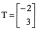and the image so obtained is enlarged by E [(O, O), 2]. Write the 3 co-ordinates of the vertices of the images so formed and represent AABC and its both images in the same graph.
23. A line segment AB joining the pointsA (4, 1) and B (7, 5) is transformed to the line segment AB' joining the points A'(-4, 1) and B'(-7, 5). Find the 2x2 matrix that represents this transformation.
24. Find the mean deviation and its coefficient from median of the data given below:
10, 50, 60, 40, 30, 2
25. Calculate the coefficient of variation from the data given below.----- 0 -----